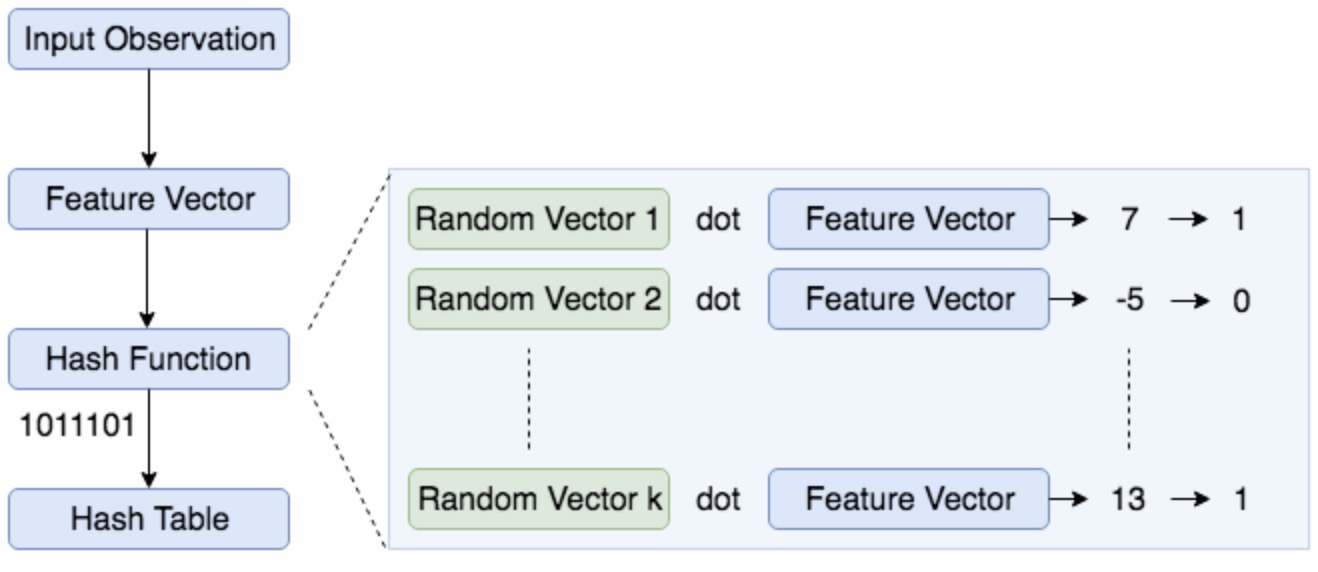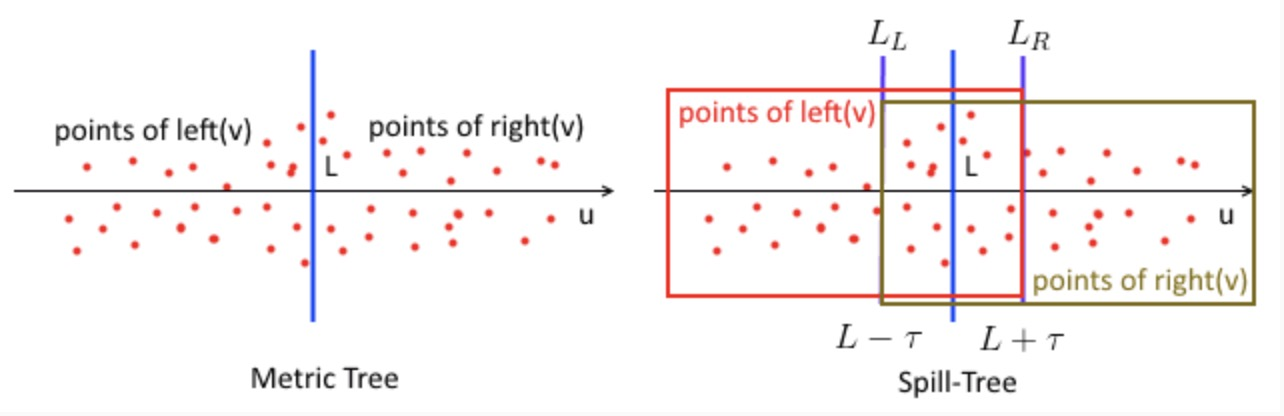KNN Algorithms

Posted by Gwan Siu on October 14, 2018

1. Introduction

Neareset neighborhood algorithm is one kind of instance-learning algorithm, which has been attracted attention by researchers because the error-rate of 1-nearest-neighbor classification is less than twice the Bayes rate. However, the efficiency of nearest neighborhood search is not satisfied in large-scale data. Over the last two decades, many significant research effort has been spent to improve its efficiency. In this article, I will introduce KNN algorithms and asymptotic analysis in Section II. In Section II, local sensitive hash algorithm is introduced to try to improve the efficiency of knn. In Section III, I will focus on tree algorithms, and improve knn efficiency in the view of data structure.

2. KNN Algorithm

2.1 KNN Algorithm

Assumed training data $D=((x_{1},y_{1}),(x_{2},y_{2}),…,(x_{n},y_{n}),…,x_{N},y_{N})$, where $x_{i}\in\mathcal{R}^{n}$ is $n$ dimensional feature, $y_{i}\in \mathbb{Y}={c_{1},c_{2},…,c_{K}}$ and $N$ and $K$ are the number of data and the number of classes respectively.

1. given a query data $(x,y)$ and distance metric $d$, distance between query point and each training data point is obtained by computing $d(x,x_{i})$ for $i=1,…,N$, and find the $k-th$ neighborhoods based on the calculated distance. Let $N_{k}(x)$ denote the neighbor containing these $k$ closest points.
2. In the neighbor $N_{k}(x)$, the class $y$ is decided according to the decision rule(e.g. voting rule):

where $w_{i}$ is weight assigned to each point in $N_{k}(x)$, and it means the weighted voting rule, but in naive KNN algorithm $w_{i}=1$.

2.2 Where does KNN comes from?

KNN algorithm is one kind of non-parametric density estimation method. It comes from parzen density estimation: $\begin{equation} \hat{p}(X)=\frac{1}{N}\displaystyle{\sum_{i=1}^{N}\kappa(X-x_{i})} \end{equation}$ where $\kappa$ is a kernel, e.g. block kernel or radius basis function kernel.

The above formulation can be written as more generally:

where $V$ denote volumn of data.

Furthermore, KNN algorithm can be shown as:

where $k-1$ means it don’t take itself into consideration, and $V(X)$ is the neighbor of $X$.

From the above analysis, the decision boundary function on KNN classifier based on bayes rule can be derived:

where $\pi_{1}=\pi_{2}$(the prior term) so that $\ln\frac{\pi_{1}}{\pi_{2}}=0$. In voting KNN classifier, pick $K_{1}$ and $K_{2}$ implicitly by picking $K_{1}+K_{2}=K,V_{1}=V_{2}$, and $N_{1}=N_{2}$.need to know more, why voting knn, k_1 + K_{2}=K, v_1=v_2

2.3 Asymptotic Analysis on KNN

Assumed test sample $X$, NN sample is denotes as $X_{NN}$ and $X\leftrightarrow I$ represents the event $X$ is class $I$. The case of nearest neighbor($k=1$) is only considered, the condition risk $r_{1}(X,X_{NN})$ can be formulated as:

when infinite samples are available, $X_{NN}$ will be so close to $X$.

The condition bayes risk: %

the procedure does not consider any information about underlying distribution and only the class of the single nearest neighbor determines the outcomes of the decision.

Thus, the asymptotic condition risk is obtained:

it can be shown that $\epsilon_{1}^{\ast}\leq 2\epsilon^{\ast}$. In fact, the error rate of neighbor classifier is less than twice bayes error rate.

2.4 The optimal K.

From the analysis above, we can see that the performance of KNN classifier is very sensitive to the choice of K. When K is decreases to 1, the decision boundary becomes more complex, which means the KNN classifier tend to overfitting, and test error becomes large because KNN classifier is sensitive to instance around its neighbor. When K tend to large, the decision boundary will become more smooth.

2.5 Distance Metric

The choice of distance is also importance for KNN algorithm. The commonly used distance metric is listed as below:

• Euclidean distance:
• Or equivalantly,
• Other metric:
• $L_{1}$ norm: $\vert x-x^{‘}\vert$
• $L_{\infty}$ norm: $\max\vert x-x^{‘}\vert$(elementwise)
• Mahalanobis: where $\Sigma$ is full, and symmetric
• Correlation
• Angle
• Hamming distance, Manhattan distance

2.6 KNN via intance-learning algorithm

• A distance metric
• How many nearby neighbors to look at?
• A weighting function
• How to relate to the local points?

3. Local Sensitive hash

3.1 Random Projection

Johnson-Lindenstrauss Lemma: The lemma states that a set of points in high-dimensional space can be embedded intto a space of much lower dimension in such a way that the distances between points are nearly preserved. This lemma is widely used in compress sensing, manifold learning, dimension reduction, and graph embedding.

Given $0<\epsilon<1$, a set of $X$ of $m$ points in $\mathbb{R}^{N}$, and a number $n>\frac{8\ln(m)}{\epsilon^{2}}$, there is a linear map $f:\mathbb{R}^{N}\rightarrow \mathbb{R}^{n}$ such that:

The formula ca be rearranged:

One proof of the lemma takes $f$ to be a suitable multiply of the orthogonal projection onto a random subspace of dimension $n$ in $\mathbb{R}^{N}$, and exploits the phenomenon of concentration of measure.

3.2 Local Sensitive Hashing in Large-scale Data

The time complexity of naive KNN algorithm is liner, $O(N)$, which is not effective in large-scale dataset. Local sensitive hashing is a hashing based algorithm to identify approximate nearest neighbors.

Local sensitive hash algorithm is a family of hash function, which can hash data points into the slots such that data points near with each other are located in the same slots with high probability, while data points far from each other are likely to be in different slots with low probability.

Assume $x_{1}$ and $x_{2}$ are two data points, $H$ is a hash function, and metric function is $d$, then

• If $d(x_{1},x_{2})$ is high when $P(H(x_{1})==H(x_{2}))$ is high.
• If $d(x_{1},x_{2})$ is low when $P(H(x_{1})==H(x_{2}))$ is low.

LSH using random projection method:

In the implementation of LSH, we firstly construct a hash table of all possible bin, which represents similary item. Each bin is a binary code(e.g. 001,101,…), such that two similar sample are likely represented by the same bin. The procedure of LSH is:

1. Create unit random vectors $r_{i}\in$ with $d$ length, for $i=1,…,k$, where $k$ denotes the number bits of binary code and $d$ is the dimension of the feature vector.
2. Given a sample $x$, we compute the dot product of unit random vector $r_{i}$ and $x$, and then assign a binary value $c_{i}$ by $h(x)=\text{sgn}(x\cdot v_{i})$.
3. Repeat step 2 k times, concatenate all binary value $c_{i}$ for $i=1,…,k$.
4. Encode all samples into hash values by repeat step 2 and step 3.
5. Group observations with same hash values together to create a LSH table.

The image below is shown the concept of LSH.4. KD-Tree

Besides hash-based methods, tree-based method is another popular method to improve efficiency of KNN. K-Dimensional tree(KD tree) is a classical tree structure, which is a binary search tree where data in each node is a K-dimensional point. KD-tree is space partition data structure for organizing points in a K-dimensional space.

4.1 The Construction of KD-Tree

Given dataset $D={x_{1},x_{2},…,x_{N}}$, where $x_{i}\in\mathbb{R}^{K}={x_{i}^{1},x_{i}^{2},…,x_{i}^{K}}]$ for $i=1,…,N$, and $K$ is the dimension of data point.

1. Selct axis of $x^{1}$, the median value $\tau^{1}$ along axis of $x^{1}$ is chosed as the threshold. Root point split $x^{1}$ dimensional space into 2 hyper-rectangle, each of which represents left child and right child of root point. The hyper-rectangle is perpandicular to the axis of $x^{1}$.
2. Recursively construct k-d tree for two subsets and split until all samples have been assigned to all nodes.

First sort the points in each dimension: $O(dn\log(n))$ time and $dn$ storage. All samples are stored in an array $A=[1,…,d,1,…,n]$.

Finding the widest spread and equally dividing into two subsets can be done in $O(dn)$ time.

Constructing the k-d tree can be done in $O(dn\log(n))$ and $(dn)$ storage.

A node has 5 fields:

• axis(splitting axis)
• value(splitting value)
• left(left structure)
• right(right structure)
• point(holds a point if left and right children are NULL)

4.2 The Search of KD-tree

We take $k=1$ as our example to find the nearest neighbor based k-d tree structure. $x$ denotes testing sample.

1. From the root point, recursively visit nodes of k-d tree(if the dimension $x^{i}$ is less than threshold, then move to left node; Otherwise, move to right node.) unitl the test sample arrives to leaf node.

2. the points in the leaf node is as closest point.
3. Recursively backtrack, in each node, 3 operations should be done: (a). If the point in the node is more closer to test sample, then the point in the node is as the closest point. (b). The cloest point must exist area corresponding to its child node, and check whether the closest point in the area of its child node. We set $B_{r}(x)$ as the ball with $r$ radius, and to check if intersection with area of node. If they has intersection, then closest point possibly exist. Otherwise, keep backtracking. (c). Until backtracking to rootpoint, the search is end, and return the closest point.

5. Ball-Tree

Ball tree is another variance of k-d tree. Ball tree is different from k-d tree, ball seperate hyper-space into several hper-sphere while k-d tree seperate hyper-space into several hyper-plane. Similar with k-d tree, ball tree has 2 chariteristics:

1. Ball tree is a binary tree, it means: nodes=sets of points, root = all points.
2. at each internal nodes, all points are partitioned into 2 disjoint sets. Each subset is disjoint with each other.

Assume $N(\nu)$ be all samples at node $\nu$, $\text{left}(\nu),\text{right}(\nu)$ are left or right child of node $\nu$.

5.1 Construction of Ball Tree

Find the median value is very expensive, thus construct ball tree by applying heuristic method, i.e. find the mean value.

1. Randomly pick $p\in \mathrm{N}(\nu)$, then let $p_{l}$ and $p_{r}$ be the point farthest from $p$ at left or right side.
2. Find mean value $\mu_{i}=\frac{p_{l}+p_{r}}{2}$ to split all points, then all points are smaller than $\mu_{i}$ go to $\text{left}(\nu)$, and all points are larger than $\mu_{i}$ go to $\text{right}(\nu)$
3. Recursively step 2 to construct ball tree until all points are assigned.

5.2 The search of Ball tree

After metric tree is constructed at each node we have:

• the decision boundary $L$, which is a $d-1$ dimensional plane orthogonal to $\mu$ that goes through $\mu$.
• a sphere ball $\mathbb{B}$ s.t. all points $\mathrm{N}(\nu)$ are in this plane. In other words, let $\text{center}(\nu)$ be the center point of $\mathrm{B}$ and $r(\nu)$ is the radius, and $\mathrm{N}(\nu)\subset \mathrm{B}(\text{center}(\nu), r(\nu))$.
1. Given a query point $q$, search in Ball tree guided by depth-fisrt search.
2. The decision boundary $L$ is used to decide the query point should be go to $\text{left}(\nu)$ or $\text{right}(\nu)$, until it arrives to left node.
3. Assume $x$ is maintained: nearest neighbor, let $d=\lVert x-q\rVert$(distance from best $x$ to the query)。
4. We can use $d$ to prune nodes: we can check if a node is good or not better than $x$. No point better than $x$ if $\lVert \text{center}(r)-q\rVert-r(r)\geq d$.

This algorithm is very efficient when the dimensionality is $\leq 30$, but it slow down when the dimensionality is $\geq 30$. In fact, ball tree can find NN very fast, and spend 95% time to construct tree. Thus, spill tree is proposed to overcome this issue.

6. Spill-Tree

Spill tree is a variant of ball tree(or metric tree). Different from ball tree(or metric tree), set of points $\text{left}(\nu)$ and $\text{right}(\nu)$ no longer gequire disjoint.

Partition strategy:

1. In ball tree, the decision boundary $L$ go through the mean value $\mu$. In spill tree, we define additionally hyperplane $L_{L}$ and $L_{R}$, where $L_{L}=L-\epsilon$ and $L_{R}=L-\epsilon$, $\epsilon$ is shared area.
2. It means that the $\text{left}(\nu)$ contains all points on the left of $\text{right}(\nu)$ and the $\text{right}(\nu)$ contains all points on the right of $\text{right}(\nu)$.

Why allowing overlap?

• Find the neighbor approximately, not exactly.6.1 The Search of Spill Tree

• Don’t trackback at all-just do a tree descent, not depth-fisrt
• Consider a case when $q$ is close to $L$: it’s true that the true NN might be on the other side of $L$
• the true NN on the over side van be catched by allowing overlap
• by varying $\tau$ we can reduce the probability of a mistake.

7. NV-Tree